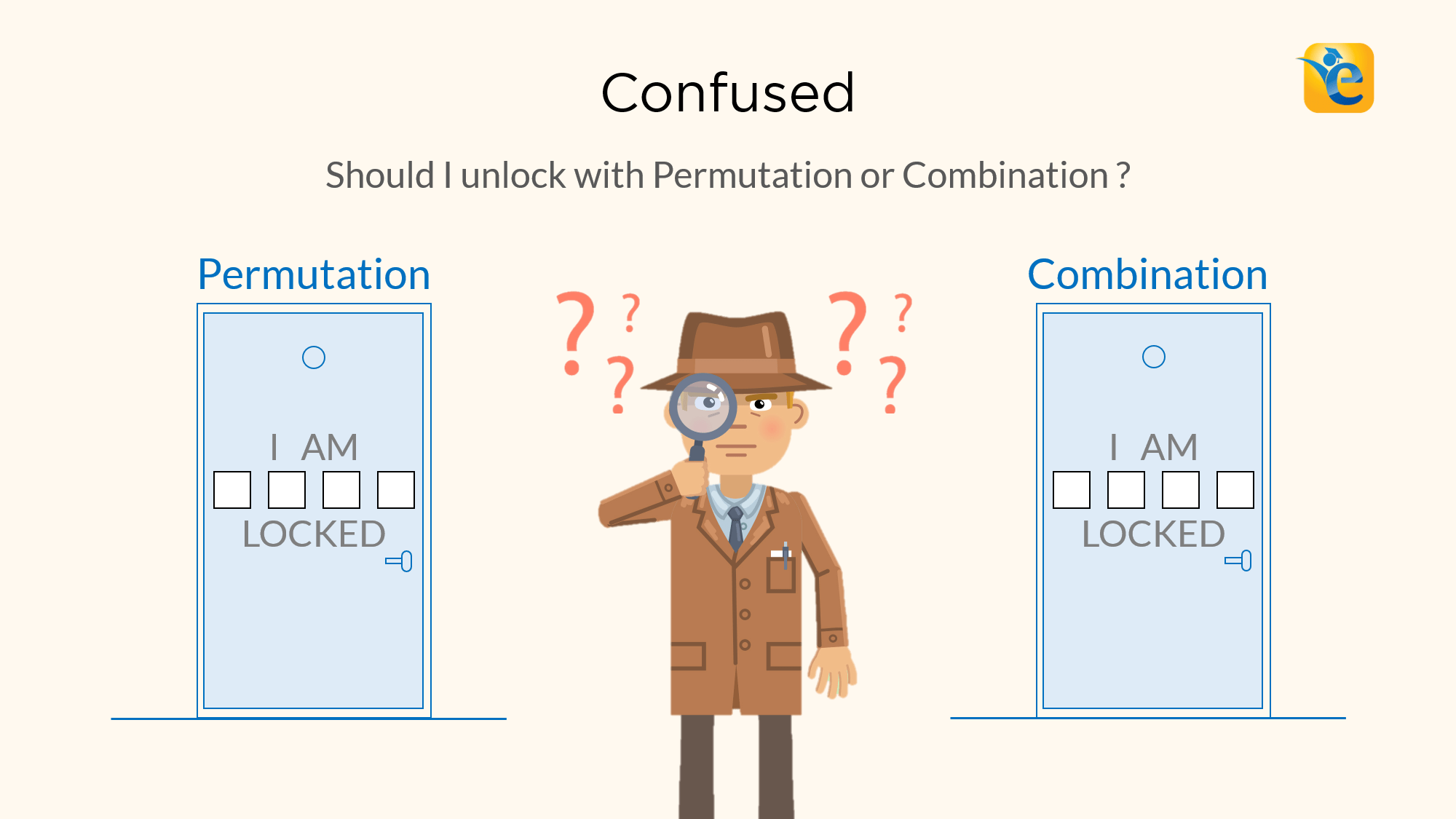# Difference between Permutation and Combination explained with examples

Permutations and Combinations is a topic full of conundrums. The biggest one is, understanding the difference between permutation and combination. Should I solve this question using permutation or combination? In this article, we will give you a foolproof method to differentiate between the two. In the last article of ‘Permutation and Combination’ series we talked

Read More# Properties of Quadrilaterals – Rectangle, Square, Parallelogram, Rhombus, Trapezium

In Euclidean geometry, a quadrilateral is a four-sided 2D figure whose sum of internal angles is 360°. The word quadrilateral is derived from two Latin words ‘quadri’ and ‘latus’ meaning four and side respectively. Therefore, identifying the properties of quadrilaterals is important when trying to distinguish them from other polygons. So, what are the properties

Read More# GMAT Quant – Tips to score a Q50+ in the GMAT Quantitative Reasoning Section

A Q50 score on the GMAT Quant section corresponds to an 85 percentile score. A Q50+ GMAT quant score does not only look good on your score report but also proves that you have the required quantitative ability to get through business school. This quantitative ability will hold you in good stead even after business

Read More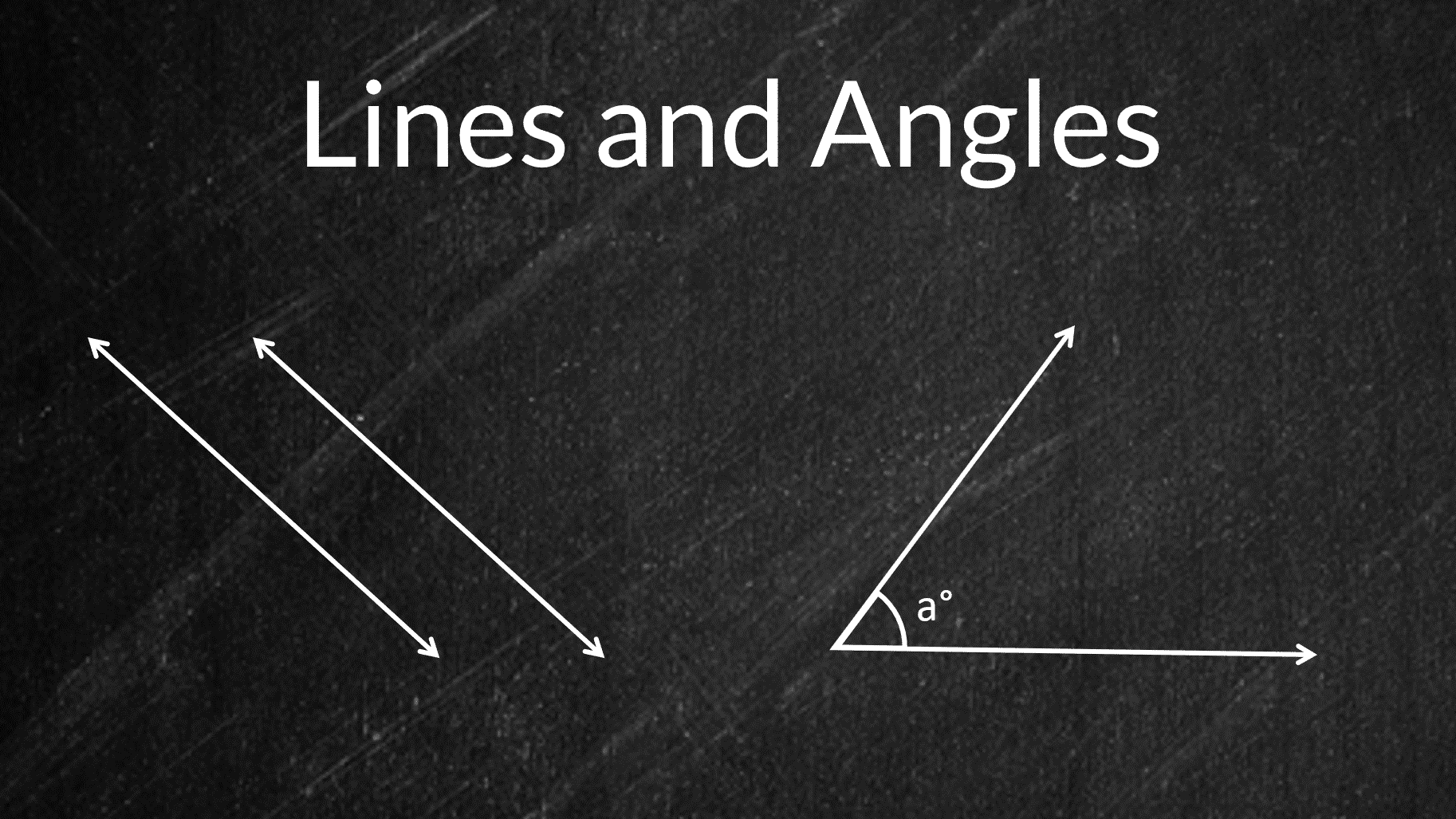# Lines and Angles – Definitions & Properties (Geometry Tutorial)

Ancient mathematicians introduced the concept of lines to represent straight objects which had negligible width and depth. Considered as a breadth less length by Euclid, lines form the basis of Euclidean geometry. When two rays(part of a straight line) intersect each other in the same plane, they form an angle. The point of intersection is

Read More# Triangles Properties and Types – Angles and Sides

In this article, we are going to learn about the simplest form of a polygon, a triangle. All polygons can be divided into triangles, or in other words, they are formed by combining two or more triangles. Thus, understanding the basic properties of triangles and their types is important. Here is an outline of the

Read More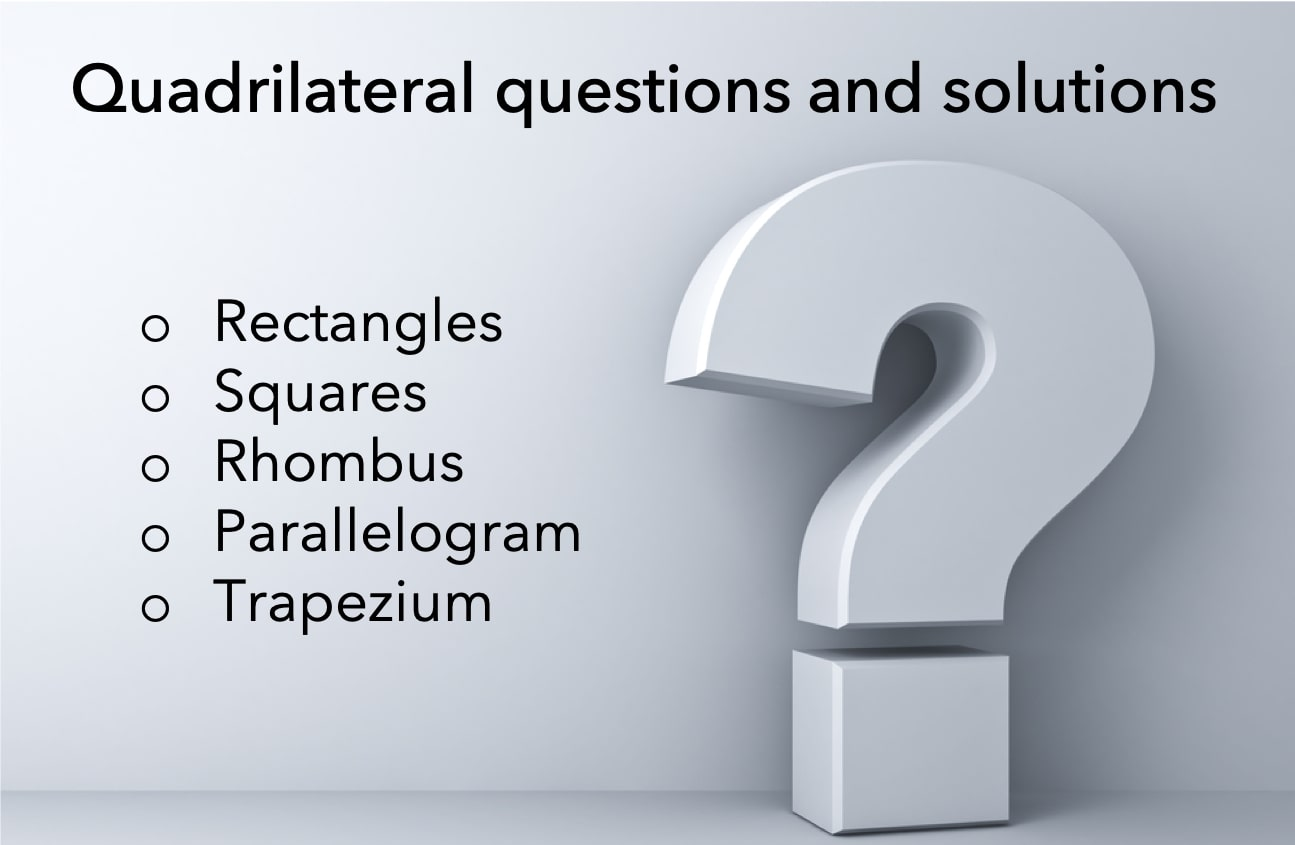Quadrilateral questions have always been a head-scratcher for a lot of candidates taking competitive or standardized exams. The primary reason behind it is learning a concept is easier as compared to applying that concept to solve questions. Therefore, to help you with the application of properties of quadrilaterals we have shared a few quadrilateral questions

Read More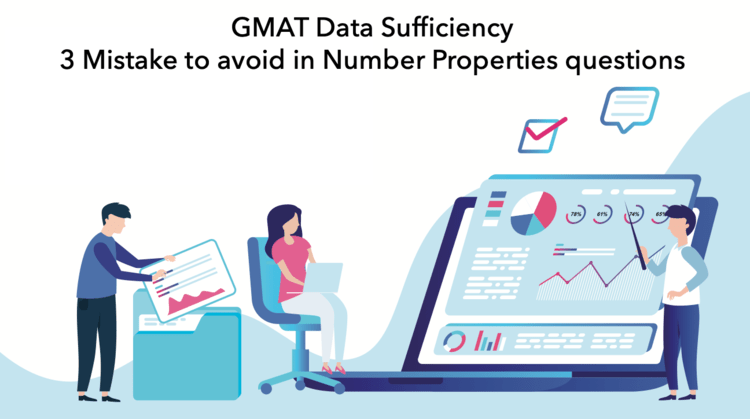# GMAT Data Sufficiency – 3 Mistake Students make in Number Properties questions

Typical GMAT data sufficiency questions are followed by information in the form of two or three statements. You have to figure out which statements you need to correctly answer the question. Many students who do reasonably well in problem-solving questions continue to struggle with GMAT data sufficiency questions. Students often realize that they couldn’t answer

Read More# GMAT preparation and MBA admissions | One Blog to rule them all

This is the place where you’ll find the legendary work of the 3 elves of middle internet. These wise elves traveled to the deepest corners of the digital space and forged this blog post with their flair for creating useful content on GMAT preparation and MBA admissions. Moreover, they work tirelessly to create new and

Read More# Introduction to GMAT Algebra – Algebraic Expressions | Linear & Quadratic Equations

Read More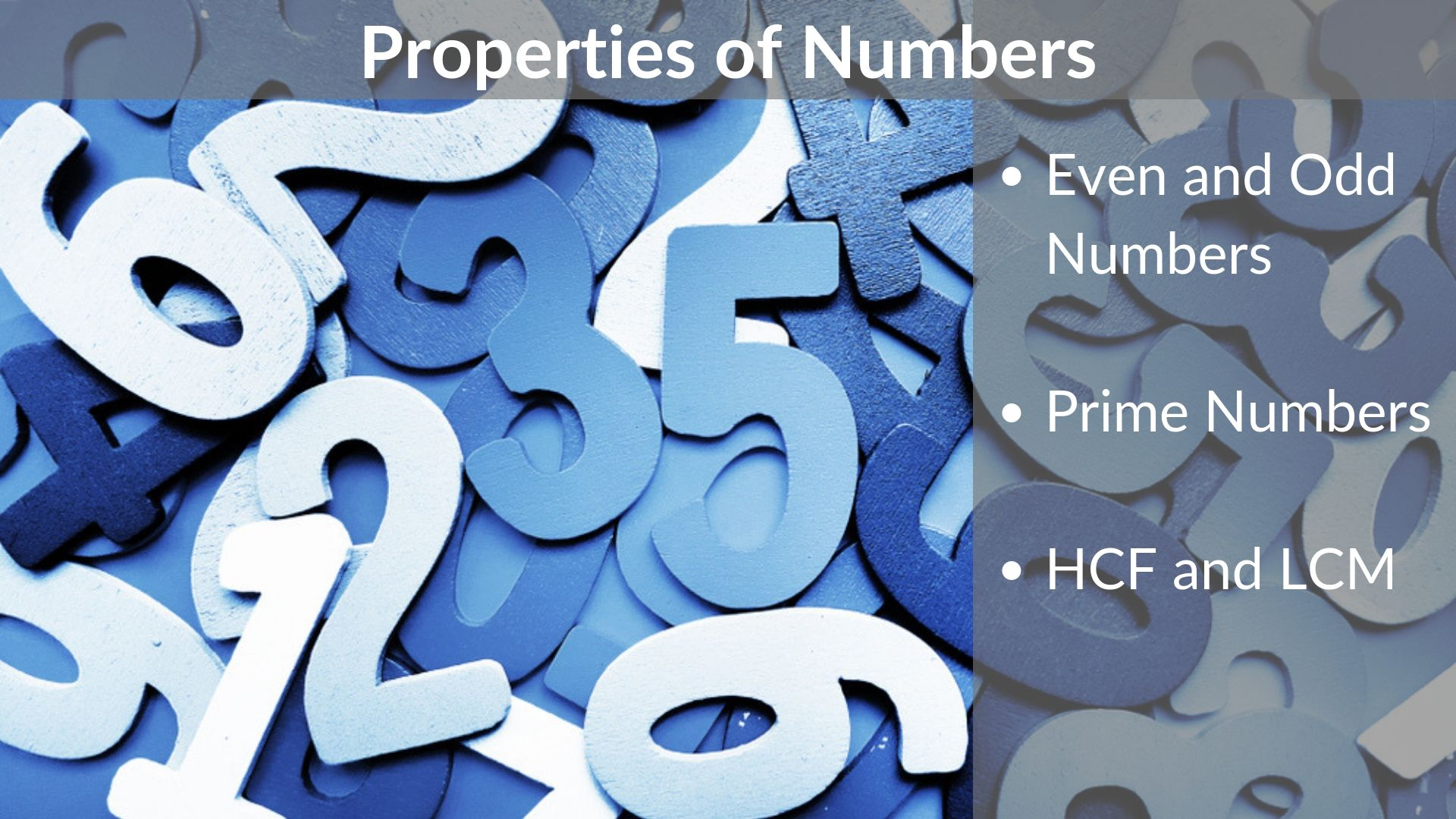Read More